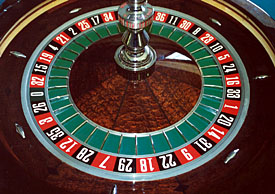# Why Casinos Don’t Lose MoneySo, how does this tie into casinos?  Let’s take the roulette wheel as our example.  There are 37 total numbers.  18 reds, 18 blacks, and 1 green.  If you guess red or black correctly, you’ll get a 1:1 payout (ie: If you bet $1, you’ll get back$2, thereby winning $1). If the wheel lands on green (0), both red and black lose. This is where the casino gets it’s edge in this particular gamble. Let’s calculate the expected value of a$1 bet on red.
$$E[X] = \frac{18}{37}(\1)+\frac{18}{37}(-\1)+\frac{1}{37}(\-1) = -\.03$$
What this means is you have an 18/37 chance of winning $1 (if it lands on red), and 18/37 chance of losing$1 (if it lands on black), and a 1/37 chance of losing $1 (if it lands on green) The expected profit for playing this game is negative 2 cents. Now, sometimes you’ll win, and sometimes you’ll lose, but if you play enough times, you’ll be averaging a loss of 23 cents per round. This is where the law of large numbers comes into play. As long as enough people are playing, the house will be averaging a profit of 2 cents for every dollar bet on that roulette table. Question: What is the expected value of correctly guessing a specific number? There are 37 numbers, but the payout is 35:1 (You get paid$35 for each dollar you bet)  Based on this answer, is it smarter to try guessing the color or guessing the number?